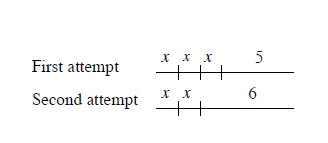### Home > MC1 > Chapter 2 > Lesson 2.4.1 > Problem2-131

2-131.

Now Cecil can slide on his tightrope!1. The first time that he crossed his new tightrope, he did three slides and walked five feet before going down the ladder. The second time, he did only two slides and then walked six feet to the end of the rope, as shown in the diagram at right. Write an equation to represent Cecil's two trips across the tightrope.

In Cecil's first attempt he crossed the tightrope like this: $x+x+x+5$ $=$ length of the tightrope

In Cecil's second attempt he crossed the tightrope like this: $x+x+6=$ length of the tightrope

Because both equations are equal to the length of a tightrope, you can make them equal to each other,
like this: $x+x+x+5=x+x+6$

Combine the $x$'s to make the equation $3x+5=2x+6$.

2. How far does Cecil travel in each slide? Show how you know.

Cecil travels $1$ foot in each slide. Remember to show how you know.

3. How long is the tightrope? How can you tell?

Three slides of one foot each and $5$ more feet would be $3(1)+5$. How long is this?